# 卡方拟合优度检验

• A+

### 分类数据的卡方拟合优度检验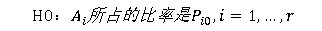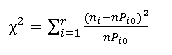mathematica实现

``````data1 = {315, 108, 101, 32};(*实际个数*)
556*(9/16) // N(*理论中的黄圆个数*)
>>312.75
556*(3/16) // N(*理论中的绿圆个数*)
>>104.25
556*(3/16) // N(*理论中的黄皱个数*)
>>104.25
556*(1/16) // N(*理论中的绿皱个数*)
>>34.75
dist1 = {312.75, 104.25, 104.25, 34.75};(*理论个数*)
(*原假设是data和dist来自同一分布*)
PearsonChiSquareTest[data1, dist1, "TestDataTable"]
(*皮尔逊卡方检验*)``````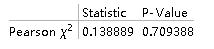### 分布的卡方拟合优度检验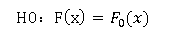mathematica实现

``````data2 = RandomVariate[PoissonDistribution, 200];
(*用泊松分布产生一组样本数据*)
Mean[data2] // N
>>3.095
(*是均值也是其最大似然估计，在缺少分布参数时可以由此来估计*)
PearsonChiSquareTest[data2, PoissonDistribution, "TestDataTable"]
(*用皮尔逊卡方检验来检验这组样本数据是否来自参数为3的泊松分布*)``````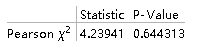``````data3 = RandomVariate[NormalDistribution[2, 3], 100];
(*用正态分布产生一组样本数据*)
H = PearsonChiSquareTest[data3, NormalDistribution[2, 3], "HypothesisTestData"];
H["TestDataTable"]
H["TestConclusion"]
>> The null hypothesis that the data is distributed according to the NormalDistribution[2,3] is not rejected at the 5 percent level based on the Pearson X^2 test.``````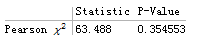• 微信公众号
• 关注微信公众号
•• QQ群
• 我们的QQ群号
•